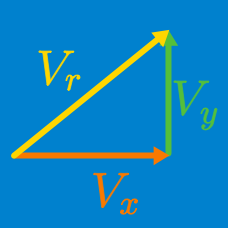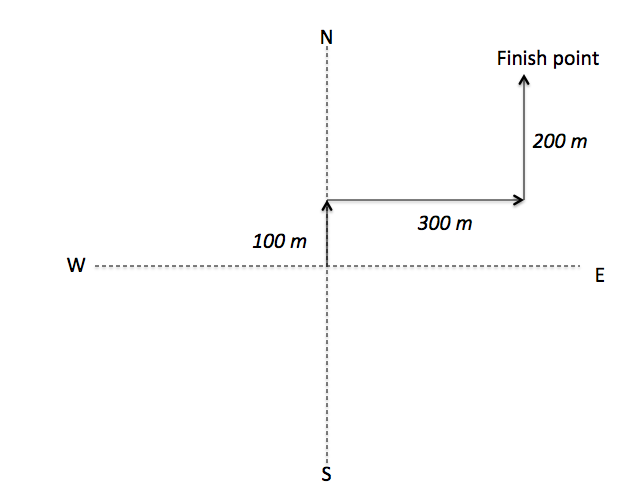Classical Mechanics

# Total displacement of a set of displacement vectors

A boy walks $8 \text{ km}$ west, then $3 \text{ km}$ south, and then $3 \text{ km}$ east. Determine his net displacement.

Let $+\hat{i}$ be the unit vector for east, and $+\hat{j}$ be that for north.Caitlyn is walking around the campus. She walks $100 \text{ meters}$ north, then $300 \text{ meters}$ east, and at last $200 \text { meters}$ north. Now, how far is she from her initial position?

Two helicopters take off from the same landing area. One helicopter proceeds $8\sqrt{2} \text{ km}$ due southwest. The second flies $4 \text{ km}$ east. Determine the final position of the second helicopter with respect to the first.

$A$ is the vector sum of the two vectors: $(5 \hat{i} - 3 \hat{j} + 2 \hat{k} )$ and $( \hat{i} + 5 \hat{j} +6 \hat{k} ).$ What is vector $A$ in unit vector notation?

A girl walks $600 \text{ m}$ east, then $1 \text{ km}$ south, and then $5 \text{ km}$ east. Find her total displacement.

$+\hat{i}$ is the unit vector for east, and $+\hat{j}$ is that for north.

×